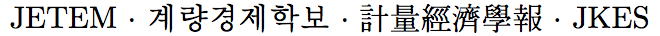Journal of Economic Theory and Econometrics: Journal of the Korean Econometric Society
Home

About this journal

Submit your article

Archive

Search

Journal of Economic Theory and EconometricsJournal of the Korean Econometric Society

• #### Dongchul Won   (College of Business Administration, Ajou University)Abstract

The paper establishes the fundamental theorem of asset pricing (FTAP) with convex transaction costs. The arbitrage pricing rules are independent of the marginal effect of transaction costs and determined by average costs for large transactions. This implies that the pricing rules are characterized by minimal information on the nature of transaction costs. Remarkably, no matter how complex the convex transaction cost functions are, the pricing rules are as simple and concrete as in the case with proportional transaction costs. This result is in sharp contrast to equilibrium pricing theory based upon the knowledge of the marginal effect of transaction costs and therefore, of transaction cost functions. Moreover, the no arbitrage condition is equivalent to viability of asset prices.

Keywords
Arbitrage Pricing, Convex Transaction Costs, Viability

JEL classification codes
G12, D52, C62
Links

KCI list
Korean ES
JETEM at SCOPUS

Powered by MathJax